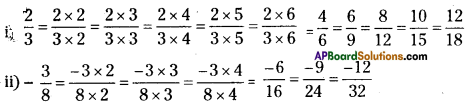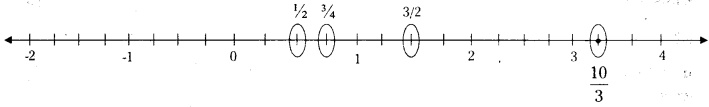# AP Board 7th Class Maths Solutions Chapter 2 Fractions, Decimals and Rational Numbers Ex 7

AP State Syllabus AP Board 7th Class Maths Solutions Chapter 2 Fractions, Decimals and Rational Numbers Ex 7 Textbook Questions and Answers.

## AP State Syllabus 7th Class Maths Solutions 2nd Lesson Fractions, Decimals and Rational Numbers Exercise 7Question 1.
Write any three equivalent rational numbers to each of the following
i) $$\frac { 2 }{ 3 }$$
ii) $$\frac { -3 }{ 8 }$$
Solution:Question 2.
What is the equivalent rational number for $$\frac { -15 }{ 36 }$$ with
(i) denominator 12
(ii) numerator 75?
Solution:
i) $$\frac{-15}{36}=\frac{-15 \div 3}{36 \div 3}=\frac{-5}{12}$$
ii) $$\frac{-15}{36}=\frac{-15 \times 5}{36 \times 5}=\frac{-75}{180}$$Question 3.
Mark the following rational numbers on the number line.
(i) $$\frac { 1 }{ 2 }$$
(ii) $$\frac { 3 }{ 4 }$$
(iii) $$\frac { 3 }{ 2 }$$
(iv) $$\frac { 10 }{ 3 }$$
Solution: